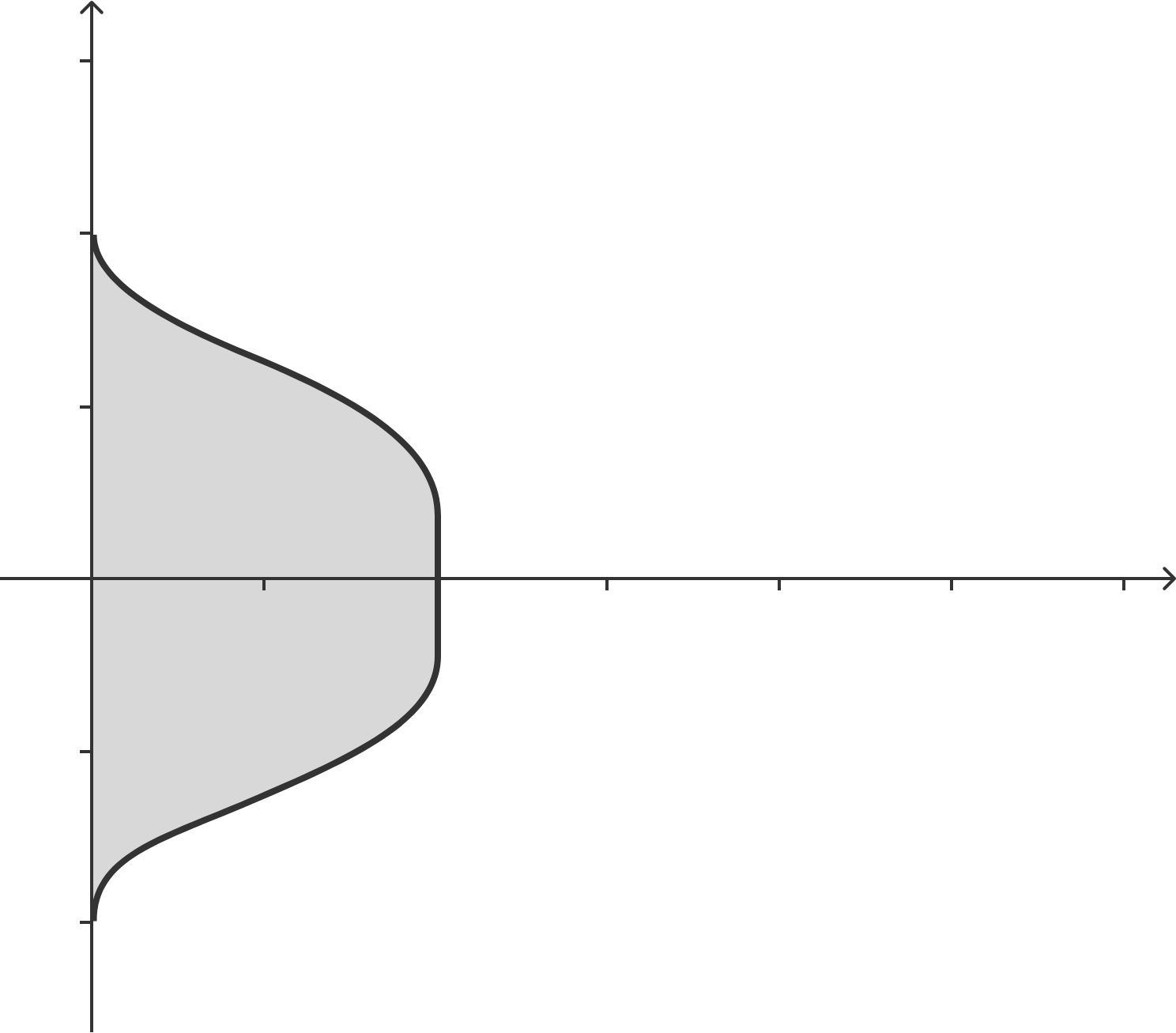# Peruvian marineraLet there be a region $R:\{(x,y) \ | \ x^{1/4}+y^{4} \leq 1\}$. What is the volume of the solid generated when $R$ is rotated around the line $x=0?$ Give your answer to 3 decimal places.


Note: You may use a calculator for the final step of your calculation.

×